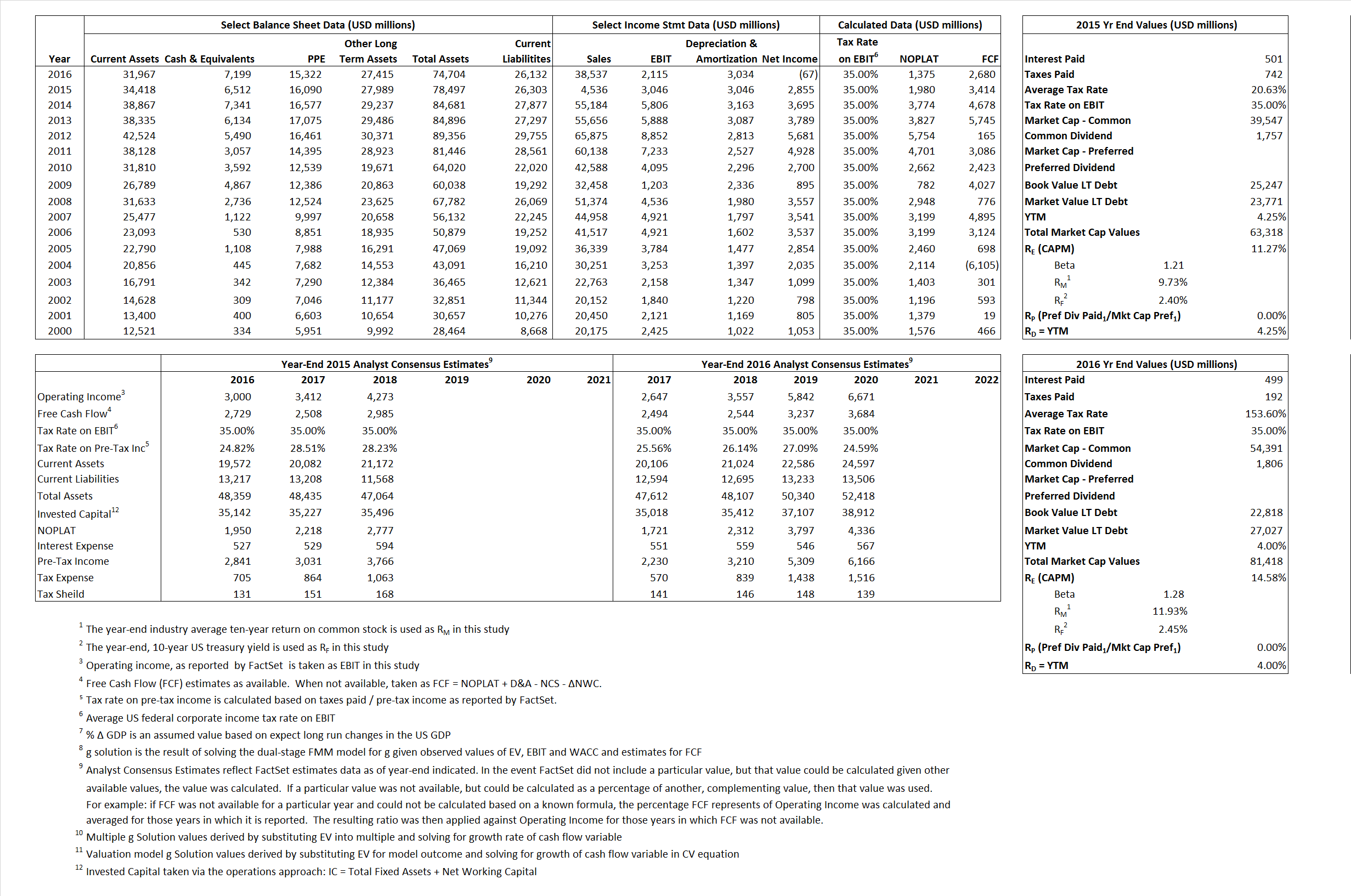# Caterpillar

## Analyst Listing

The following analysts provide coverage for the subject firm as of May 2016:

 Broker Analyst Analyst Email Wells Fargo Securities Andrew M. Casey andrew.casey@wellsfargo.com Evercore ISI David Raso david.raso@evercoreisi.com Longbow Research Eli Lustgarten elustgarten@longbowresearch.com Credit Suisse Jamie Cook jamie.cook@credit-suisse.com BMO Capital Markets Joel Tiss joel.tiss@bmo.com William Blair Lawrence T. De Maria ldemaria@williamblair.com Atlantic Equities Richard Radbourne r.radbourne@atlantic-equities.com RBC Capital Markets Seth Weber seth.weber@rbccm.com Jefferies Stephen Volkmann svolkmann@jefferies.com

## Primary Input Data## Derived Input Data

### Equational Form

Net Operating Profit Less Adjusted Taxes NOPLAT 1,980 1,375$NOPLAT\, =\, EBIT\, x\, (1 \,-\, Avg \,\,Tax\,\, Rate\,\, on\,\, EBIT)$
Free Cash Flow FCF 3,414 2.680$FCF\,=NOPLAT\,+\,Non-Cash\,Expenses-\Delta NWC\,-\,NCS$
Tax Shield TS 103 766$TS\,=\,Interest\,\,Paid\,\,x\,\, Avg \,\,Tax\,\,Rate\,\, on\,\, Pre-Tax\,\, Income$
Invested Capital IC 52,194 48.572$IC\,=\,Fixed\,\,Operating\,\,Assets\,\,+\,\,Net\,\, Working\,\, Capital$
Return on Invested Capital ROIC 3.79% 2.83%$ROIC\,=\,\frac { NOPLAT }{ IC }$
Net Investment NetInv (1,564) (588)$NetInv\,=\,{ {IC}_{1}}-{{IC}_{0}}+Depreciation$
Investment Rate IR -78.99% -42.77%$IR\,=\,\frac {NetInv}{NOPLAT}$
Weighted Average Cost of Capital
WACCMarket 8.30% 9.03%$WACC\,=\,\frac { E }{ V } { R }_{ E }\,+\,\frac { P }{ V } { R }_{ P }\,+\,\frac { D }{ V } { R }_{ D }\left( 1- Avg\,\, Tax\,\,Rate\,\,on\,\,Pre-Tax\,\,Income \right)$
WACCBook    6.43%  6.86%
Enterprise value
EVMarket 56,806  74,219$EV\,=\,Market\,\,Cap\,\,Equity\,+\,\,Long\,\,Term\,\,Debt\,-\,Cash$
EVBook  58,282  70,010
Long-Run Growth
g = IR x ROIC
-3.00%  -1.21% Long-run growth rates of the income variable are used in the Continuing Value portion of the valuation models.
g = %$\Delta$ GDP   2.50%  2.50%
Margin from Operations M  67.15%  5.49%$M\,\,=\,\,\frac{EBIT}{SALES}$
Depreciation/Amortization Rate D  50.00%  58.92%$D\,\,=\,\,\frac{D+A}{EBITDA}$

## Valuation Multiple Outcomes

The outcomes presented in this study are the result of original input data, derived data, and synthesized inputs.

### model g solution

12/31/2015 12/31/2016 12/31/2015 12/31/2016 12/31/2015 12/31/2016

EV/SALES$\frac {EV}{Sales} \,= \,\frac{ROIC\, -\, g}{ROIC\,(WACC\,-\,g)}\,(1\,-\,T)\,(M)$

12.52  1.93  59.36%  20.78%  29.58%  14.28%

EV/EBITDA$\frac {EV}{EBITDA} \,= \,\frac{ROIC\, -\, g}{ROIC\,(WACC\,-\,g)}\,(1\,-\,T)\,(1\,-\,D)$

9.32 14.41 59.36% 20.78% 29.58% 14.28%

EV/NOPLAT$\frac {EV}{NOPLAT} \,= \,\frac{ROIC\, -\, g}{ROIC\,(WACC\,-\,g)}$

28.69 53.99 59.36% 20.78% 29.58% 14.28%

EV/FCFOPS$\frac {EV}{FCF_{OPS}} \,= \,\frac{ROIC\, -\, g}{ROIC\,(WACC\,-\,g)}\,(1\,-\,T)$

16.64 27.69 59.36% 20.78% 29.58% 14.28%

EV/EBIT$\frac {EV}{EBIT} \,= \,\frac{ROIC\, -\, g}{ROIC\,(WACC\,-\,g)}\,(1\,-\,T)$

18.65 35.09 59.36% 20.78% 29.58% 14.28%

EV/IC$\frac {EV}{IC} \,= \,\frac{ROIC\, -\, g}{WACC\,-\,g}$

1.09 1.53 59.36% 20.78% 29.58% 14.28%